##### Managerial Economics For Dummies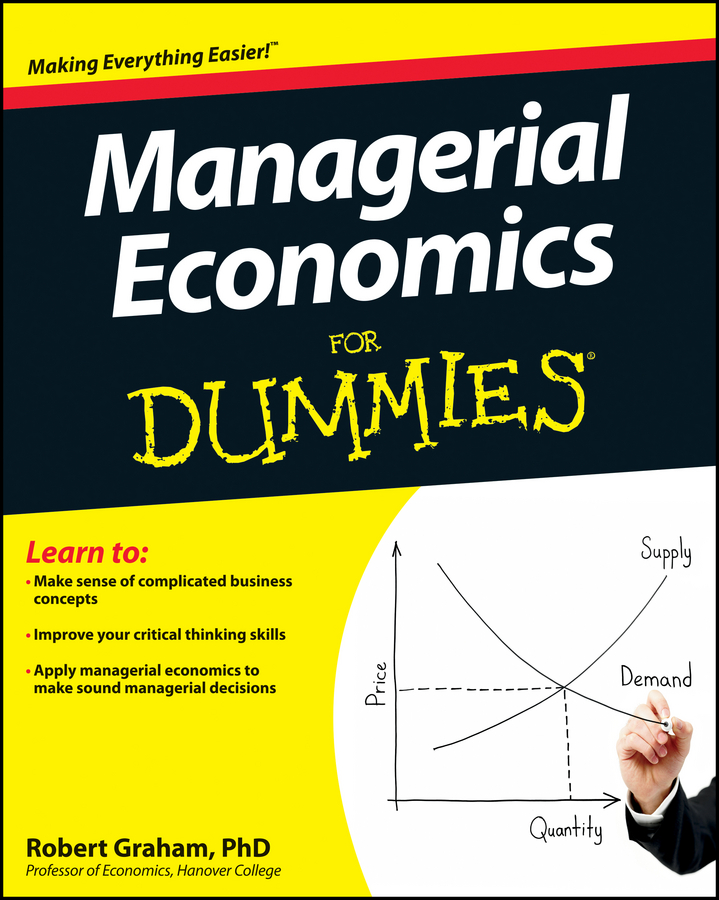Production functions typically have more than one input; however, in the case of a single input production function, you assume that the quantity employed of only one input can be varied. In other words, you have one variable input and all other inputs in the production process are fixed inputs — and you can’t change the quantity of the fixed inputs.

You’re a farmer trying to decide how much land you’re going to plant in corn and how much you’ll leave for hay. You’ve decided to treat the quantities of labor (you’re the only one working) and machinery employed constant. In this situation, labor and capital (the machinery) are fixed inputs and land is a variable input.

The following equation describes the relationship between the amount of land you use and the quantity of corn produced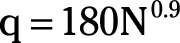Where q is the number of bushels of corn grown and N is the amount of land used.

Number of Acres of Land (N) Number of Bushels of Corn (q)
50 6,086
99 11,255
100 11,357
150 16,359
200 21,193
250 25,907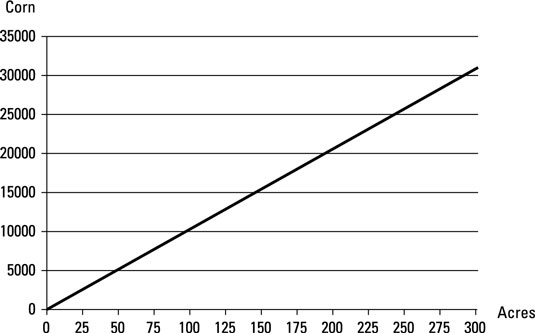## The difference between average product and marginal product

You can examine the relationship between inputs and output in a number of different ways. You may be concerned with only the total amount of corn grown — what economists call total product.

Alternatively, you may be interested in the average quantity of corn produced per acre of land, what farmers call yield, and economists call average product, or you may be interested in how much corn output increases when you plant one more acre, marginal product to economists. Each of these relationships is important, and each offers considerable insight into the production process.

• Total product refers to the entire quantity of output produced from a given set of inputs. In the equation, q represents total product. Therefore, if you plant 100 acres of corn, total product equals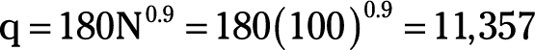or 11,357 bushels of corn produced.

• Average product refers to the output per unit of input. For a production function that has a single variable input, average product equals the total product divided by the quantity of input used. Therefore,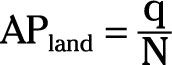If you plant 100 acres of corn, the average product equals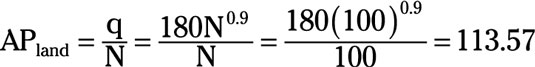or 113.57 bushels per acre.

• Marginal product is the change in total product that occurs when one additional unit of a variable input is employed. The marginal product of the 100th acre of land is 102 bushels of corn. The total product for 99 acres is 11,255 bushels, and the total product for 100 acres is 11,357 bushels. Thus, the difference or change between the two is 11,357 – 11,255 or 102 bushels of corn.

Marginal product is also determined with calculus.

To determine marginal product with calculus, take the following steps:

1. Take the derivative of total product with respect to the input. In the example, this is land (N).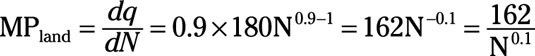2. Substitute in the appropriate value for the input. So, to determine the marginal product of the 100th acre of land, substitute 100 for N and solve (with the help of your computer or calculator).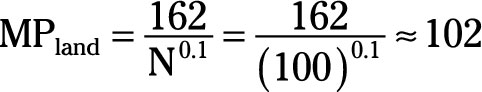Thus, if you start at 100 acres, adding another acre of land increases output by 102 bushels.

## Diminishing returns

The law of diminishing returns states that ceteris paribus, as the quantity employed of an input increases, eventually a point is reached where the marginal product of an additional unit of that input decreases.

The term ceteris paribus indicates that all other things — for example, the quantities employed of other inputs and technology — are held constant. Therefore, the law of diminishing returns indicates that after some point, additional units of a variable input aren’t as productive as preceding units of the input.